Learn US

# Greatest Integer Function

5.2k views

 1 Introduction 2 Greatest Integer Function 3 Domain and Range of greatest integer function 4 The Inverse of Greatest Integer Function 5 How to Graph Greatest Integer Function 6 Vertical and Horizontal shift in Greatest Integer Function graph 7 Summary

1 October  2020

## Introduction

Do you know, there is a family of functions which returns only integers based on a certain rule, corresponding to a real number. Greatest integer function (also called floor function) and least integer function (also called ceiling function) form a part of specific types of functions which result in only an integer. In this blog, we will study about Greatest Integer Functions.

### What is a function?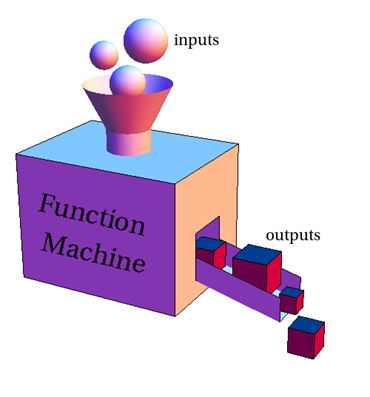A function is a kind of machine which takes an input and produces an output.

Let’s say f(x) = 2x + 3, If you substitute x = 2 you will get

f(x) = 2(2) + 3 = 4 + 3 = 7.

For x = -3 you will get

f(x) = 2(-3) + 3 = -6  + 3  = -3.

So f(x) = 2x + 3 is a function which is taking different values of x called input and results in various values of f(x) called output.

Even the formulas that we apply can be treated as functions because they depend on one or more variables for instance volume of water in glass depends on the radius of the container and the height to which it is filled. You keep on changing the values of radius and height and you get different values of the volume.There are various types of functions depending on the power or form of the variable involved in it. One specific function is greatest integer function.

## Greatest Integer Function

Let’s understand about greatest integer functions from the calculation of income taxes. Each income tax table inputs your income and outputs your tax, and that's a function. If your income is not listed in the table then you can use an explicit continuous formula instead (the formula is also piece-wise linear. The slopes of all pieces depend on your income bracket, higher the brackets higher will be the slopes). If your income tax computation is automated by some software application, it uses the same function.

The most common example of the Greatest Integer Function is the Post Office system. Do you know the postage is paid based on weight…. For example, between 1 (including 1) and 2 ounces, you might pay $1.25 postage. However, when you hit 2 ounces to almost 3 ounces you pay$2.00. This progression is a greatest integer function and is currently used by the Post Office system. You could probably get their rate chart and see.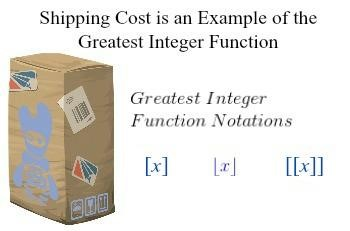### What is greatest integer function?

The greatest integer function is denoted by f(x) = [x] and is defined as the greatest integer less or equal to x. This means if you take x as an integer then the answer will be that integer, whereas if you take x = non-integer then it will give an answer which is the integer just before x.

###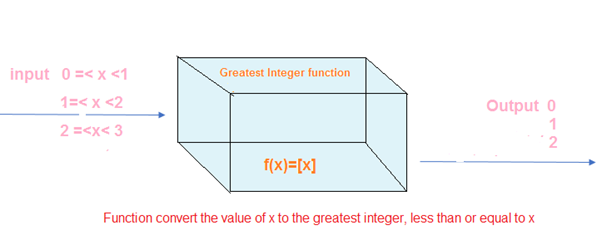Example 1

When we write [2.5], it stands for the greatest integer less or equal to 2.5.

### Solution:-

There are infinite integers less than or equal to 2.5.

2, 1, 0, -1, -2, …… are all less than 2.5

Out of all of these 2 is the largest integer so that becomes the answer for [2.5].

So we notice that the number we are looking for has to meet two conditions:

•     The number has to be an integer.
•     It has to be the greatest of all integers which are smaller than or equal to 2.5.

From the above points, we conclude that [2.5] = 2.

 Example 2

What is the greatest integer value of  -1.3?

### Solution:-

For the greatest integer value of negative numbers we usually make a mistake. It seems that the value of [-1.3]  = -1.

But it is wrong…….I am sure you wonder why so. Let us understand this on the number line that follows:-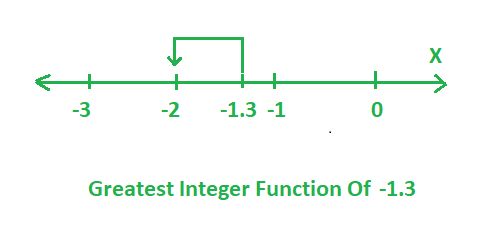So you see, that the integer just before -1.3 is -2 and not -1. Therefore,

[-1.3] = -2.

## Domain and Range of greatest integer function

The collection of all x values which can be substituted in a function is called its domain and the collection of all corresponding values of f(x) is called the range of the function. From the graph, we can see that when any real number is substituted in the greatest integer function it results in the largest of all the smaller integers.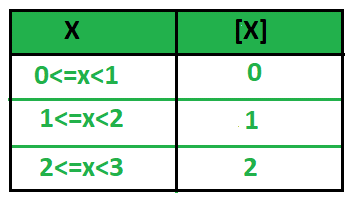Even from the above graph, we see that x can take any value from the x-axis but y only can take integral values.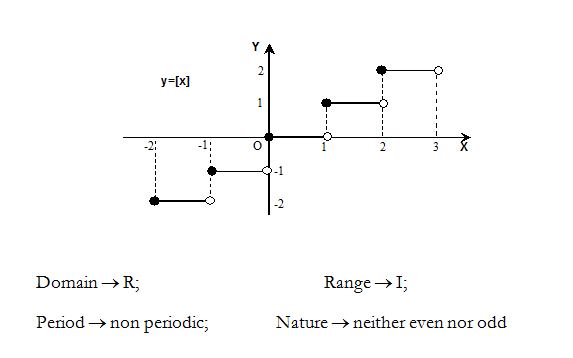So the domain of greatest integer function is Real numbers and its range is Integers.
For f(x)=[x],Df= R   and Rf=  Z.

We also see from the graph that it is not repeating values after any interval so the greatest integer function is non-periodic.
And also  neither f(x)≠-f(-x)  nor f(x)  ≠f(-x) for all values of f(x) so greatest integer function is neither an odd function nor an even function.

## The inverse of greatest integer function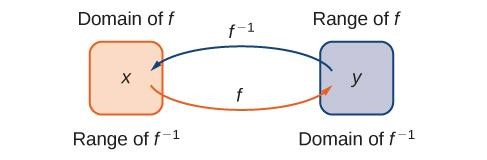The inverse of a function is a reverse definition of a function from the second set to the first set.

If there is a function defined as f: R - > R as f(x) = 4x + 3.

Then it has an inverse given by f-1 (x)=  (x-3)/4 .

A function is invertible when it is both one-one and onto. For checking the one-one function, we take two different values of x from the domain and check if they are connected to different y from codomain.

Here the function is one-one.

For checking if the function is onto or not, we find the range of the function and check if it is equal to codomain or not. In the above function, the range is R and hence it is onto also. And hence the inverse of function exists.

Let us take another example of a polynomial function defined as

f: R -> R as f(x) = x3+x2-x+2,

If you substitute any value for x from the set of real numbers (domain) the value of f(x) will always exist in reals and it will be different from the previous answer. So we conclude that f(x) defined above is both one-one and onto and hence, its inverse will exist. But every function is not invertible.

Now, let us check for greatest integer function. For the greatest integer function find out [1.2] and [1.7], which means the greatest integer value of 1.2 and 1.7. We see that both will result in 1 as the answer. So in the arrow diagram, both will be connected to the same answer so we clearly can say that the greatest integer function is not one-one. And since it is not one-one it is not invertible also. So the greatest integer function is not invertible.

## How to graph greatest integer function?

The greatest integer function, also called step function, is a piecewise function whose graph looks like the steps of a staircase.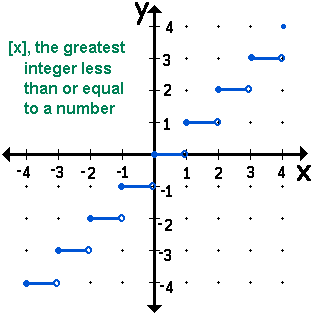So you can see that the graph of greatest integer function looks like a staircase (or steps). This is why the greatest integer function is also called a step function. But how to graph greatest integer function which is not the basic [x]. That is where the horizontal and vertical shift of the graph comes into place.

## Vertical and Horizontal shift in Greatest integer Function graph

Let us move on to the transformation of greatest integer function graph. We have seen the graph of f(x) = [x] above. Now what happens to the greatest integer function graph when we have another function as following:-

f(x) = [x-2]

 x 0 1 2.5 2.6 2.9 -1.1 -1.6 -1.9 x-2 -2 -1 0.5 0.6 0.9 -3.1 -3.6 -3.9 f(x) -2 -1 0 0 0 -4 -4 -4

And the sketch will come as follows.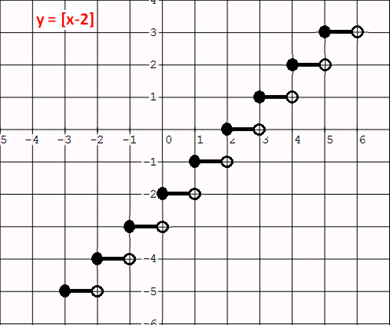Example 3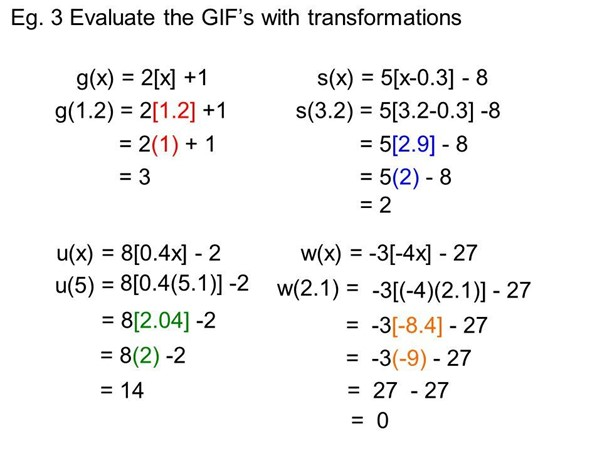Example 4

How to graph greatest integer function f(x) = [ x] + 3.

### Solution:-

 x 0 0.5 0.6 0.9 1 -1.1 -1.5 -1.9 [x] 0 0 0 0 1 -2 -2 -2 f(x) 3 3 3 3 4 1 1 1

Plotting the points on the graph we get the following greatest integer function graph:-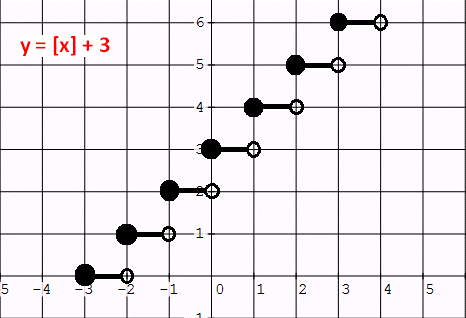Example 5

Sia hired an auto-rickshaw. The driver has set a fixed initial cost of $3 and after that, the cost depends on the distance traveled. Suppose the rickshaw driver charges$1 up to each mile then find the cost Sia has to pay while traveling 7.8 miles.

### Solution:-

The above problem can be framed as the greatest integer function.

Cost function f(x) can be written as

f(x) = [x] + 3.

We can calculate the cost of her travel at any point using the following table. The above plot can be used to understand the problem well.

 x 0.1 0.9 2.1 3.4 5.6 7.8 [x] 0 0 2 3 5 7 f(x) 3 3 5 6 8 10

That means after traveling 7.8 miles Sia has to pay \$10

## Summary

So what we have learned. Let’s recap.

Greatest Integer function is a piece-wise function also called step function because of its graph. It results in an integer equal to or less than the substituted value.

The domain of greatest Integer is R and its range is Z. Greatest integer function is not invertible.

 Example 1

 = 1      [1.5] = 1      [3.7] = 3      [4.3] = 4

BUT Beware!    [-2] = -2      [-1.6] = -2      [-2.1] = -3      [-5.5] = -6

 Example 2

Match the following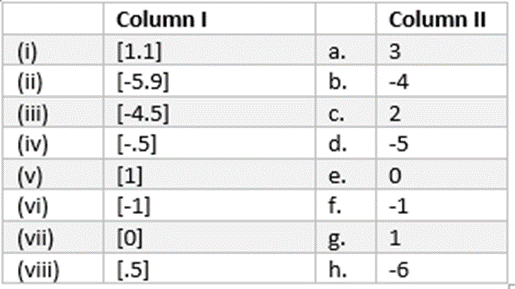### Solution:-

(i) -> g, (ii) -> h, (iii) -> d, (iv) -> f, (v) -> g, (vi) -> f, (vii) -> e, (viii) -> e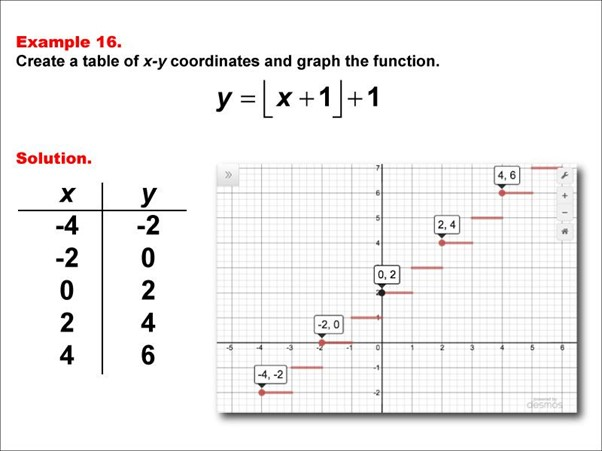GIVE YOUR CHILD THE CUEMATH EDGE
Access Personalised Math learning through interactive worksheets, gamified concepts and grade-wise courses